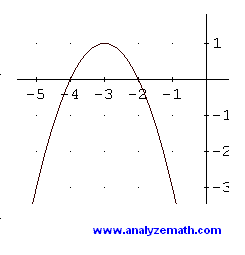# Derivative, Maximum, Minimum of Quadratic Functions

Differentiation is used to analyze the properties such as intervals of increase, decrease, local maximum, local minimum of quadratic functions.

## A - Quadratic Function in General form

Quadratic functions in their general form are written as
f(x) = a x 2 + b x + c

where a, b and c are real numbers such that a not equal to zero.
The first
derivative of f is given by
f ' (x) = 2 a x + b
Let us analyze the sign of f' and hence determine any maximum or minimum point and the intervals of increase and decrease. f '(x) is positive if
2 a x + b > 0
add -b to both sides of the inequality to obtain 2 a x > -b
We now need to consider two cases and continue solving the inequality above.

### case 1: coefficient a > 0

We divide both sides of the inequality by 2 a and obtain
x > - b / 2a
We now use a table to analyze the sign of f ' and whether f is increasing over a given interval.The quadratic function with a > 0 has a minimum point at (-b/2a , f(-b/2a)) and the function is decreasing on the interval (-infinity , -b / 2a) and increasing over the interval (-b / 2a , + infinity).

### case 2: coefficient a < 0

We divide both sides of the inequality by 2 a but because a is less than 0, we need to change the symbol of inequality
x < - b / 2a
We now analyze the sign of f ' using the table belowThe quadratic function with a < 0 has a maximum point at (-b/2a , f(-b/2a)) and the function is increasing on the interval (-infinity , -b / 2a) and decreasing over the interval (-b / 2a , + infinity).

## B - Quadratic Function in Vertex form

Quadratic functions in their vertex form are written as
f(x) = a (x - h) 2 + k

where a, h and k are real numbers with a not equal to zero.
The first derivative of f is given by
f '(x) = 2 a (x - h)
We analyze the sign of f' using a table. f '(x) is positive if
a (x - h) > 0
We need to consider two cases again and continue solving the inequality above.

### case 1: coefficient a > 0

We divide both sides of the inequality by a and solve the inequality
x > h
The table below is used to analyze the sign of f '.The quadratic function with a > 0 has a minimum at the point (h , k) and it is decreasing on the interval (-infinity , h) and increasing over the interval (h , + infinity).

### case 2: coefficient a < 0

We divide both sides of the inequality by a but we need to change the symbol of inequality because a is less than 0.
x < h
We analyze the sign of f ' using the table belowThe quadratic function with a < 0 has a maximum point at (h , k) and the function is increasing on the interval (-infinity , h) and decreasing over the interval (h , + infinity).

### Example 1

Find the extremum (minimum or maximum) of the quadratic function f given by
f(x) = 2 x 2 - 8 x + 1

### Solution to Example 1

• We first find the derivative
f ' (x) = 4 x - 8
f ' (x) changes sign at x = 8 / 4 = 2. The leading coefficient a is positive hence f has a minimum at (2 , f(2)) = (2 , -7) and f is decreasing on (-infinity , 2) and increasing on (2 , + infinity). See graph below to confirm the result obtained by calculations.### Example 2

Find the extremum (minimum or maximum) of the quadratic function f given by
f(x) = - (x + 3) 2 + 1

### Solution to Example 2

• The derivative is given by
f '(x) = - 2 (x + 3)
f '(x) changes sign at x = -3 . The leading coefficient a is negative hence f has a maximum at (-3 , 1) and f is increasing on (-infinity , -3) and decreasing on (-3 , + infinity). See graph below of f below.### Exercises on Properties of Quadratic Functions

For each quadratic function below find the extremum (minimum or maximum), the interval of increase and the interval of decrease.
a) f(x) = x
2 + 6 x
b) f(x) = -x
2 - 2 x + 3
c) f(x) = x
2 - 5
d) f(x) = -(x - 4)
2 + 2
e) f(x) = -x
2

a) minimum at (-3 , -9)
decreasing on (-infinity , -3)
increasing on (-3 , + infinity)
b) maximum at (-1 , 4)
increasing on (-infinity , -1)
decreasing on (-1 , + infinity)
c) minimum at (0 , -5)
decreasing on (-infinity , 0)
increasing on (0 , + infinity)
d) maximum at (-4 , 2)
increasing on (-infinity , -4)
decreasing on (-4 , + infinity)
e) maximum at (0 , 0)
increasing on (-infinity , 0)
decreasing on (0 , + infinity)

### More on applications of differentiation

applications of differentiation